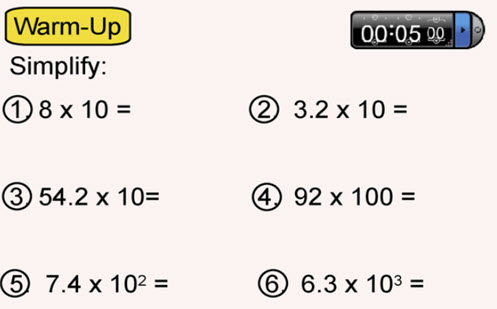Go to website

# Introduction to scientific notation

In this series of lessons, students are introduced to the concept of expressing numbers in standard or scientific form. They go on to explore how to operate with and problem-solve numbers in scientific form.

Year level(s) Year 9
Audience Teacher
Purpose Student task, Teaching resource, Teaching strategies
Format Web page
Teaching strategies and pedagogical approaches Explicit teaching
Keywords Scientific notation, Power, Index number

## Curriculum alignment

Curriculum connections Numeracy, Critical and creative thinking
Strand and focus Measurement
Topics Using units of measurement, Time, Indices
AC: Mathematics (V9.0) content descriptions
AC9M9M02
Solve problems involving very small and very large measurements, time scales and intervals expressed in scientific notation

AC9M9M03

Solve spatial problems, applying angle properties, scale, similarity, Pythagoras' theorem and trigonometry in right-angled triangles

Numeracy progression Multiplicative strategies (P10)
Measuring time (P7)
Number and place value (P10)
Proportional thinking (P7)
Understanding geometric properties (P7)
Understanding units of measurement (P10)